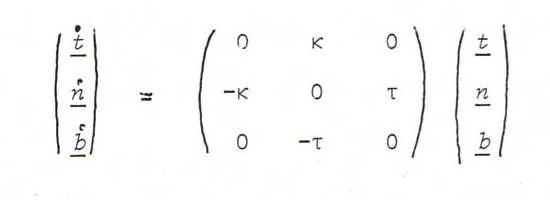### DIFFERENTIAL GEOMETRY COURSE

4. SERRET AND FRENET

Definition 30: The osculating plane (kissing plane) in a point x(t0) of the curve x(t) is the limit of the position of the plane through the tangent in x(t0) and a neighbour point x(t0+Δt) on the curve, when Δt→0.

Proposition 31: The osculating plane in x(t0) is the plane with parameter representation x(t0)+λx'(t0)+μx''(t0) and equation det(x-x(t0),x'(t0),x''(t0)=0.

Prove: Since x(t0+Δt)-x(t0)=x'(t0)+(1/2)x''(t0)Δt2 (+ terms of higher degree), the equation det(x-x(t0),x'(t0),x(t0+Δt)-x(t0)) = 0 becomes, when Δt→0, the equation det(x-x(t0),x'(t0),x''(t0)=0.

Definition 32: We call a point x(s0) on a curve x(s) whose parameter s is arc length, an inflection point of the curve if κ(s) changes sign in s0 (then the local curvature vector is 0).

Proposition 33: In the inflection points of the curve x(t), the vectors x' and x'' have either the same direction or opposite directions.

Prove: According to problem 20 we find : x .. = 0x'⊗x'' = 0.

Problem 34: Determine the equation of the osculatiom plane in an inflection point.

Problem 35: Give the equation of the osculating plane in a point x(t) of the cubic parabola from example 11 iii).

Definition 36: (Serret-Frenet frame)
Let x(s) be a curve whose parameter s is arc length. Let t(s) := x .(s) be the tangent of length 1. Choose the principal normal vector n(s) with length 1, having the same or opposite direction as the curvature vector x ..(s), as we discussed in the previous section.
So the osculating plane is x(s)+λt(s)+μn(s).
Start with t(s),n(s) and complete the frame with the so-called binormal vector b(s) so as to get a right orthonormal frame (that is to say: b := tn). Then we call t,n,b The Serret-Frenet frame.
We call the plane x(s)+λn(s)+μb(s) the normal plane, and the plane x(s)+λt(s)+μb(s) the rectifying plane.

Problem 37: Determine the equations of these three planes in an arbitrary point of the cubic parabola.

Proposition 38 (Theorem of Serret and Frenet):
There exist functions κ(s) and τ(s) withProve: The function κ is the curvature function, and we discussed the formula t . = κn in the previous section.
According to problem 9b), n is perpendicular to n ., so according to problem 8 there exist ρ and τ such that n . = ρtb. Again according to problem 8, we have ρ=n ..t.
Using n.t=0 we see by differentiation n ..t+n.t . = 0, so ρ = n ..t = -n.t . = -κ.
Likewise: since b is perpendicular to b ., we have b . = αtb.
Then α = b ..t = -b.t . = 0, and β = b ..t = -b.n . = -τ.

Definition 39: The function τ from the theorem of Serret and Frenet is called the torsion of the curve.

Proposition 40: κ2τ = det(x .,x ..,x ...).

Prove: Using x .. = κn we find x ... = κ .n + κn . = κ .n + κ(-κtb), so
det(x .,x ..,x ...) = det((1,0,0),(0,κ,0),(-κ2.,κτ)) = κ2τ.

Problem 41: Prove that det(x',x'',x''') = ||x'||6det(x .,x ..,x ...), and deduce:

τ = det(x',x'',x''')/(x'⊗x'')2